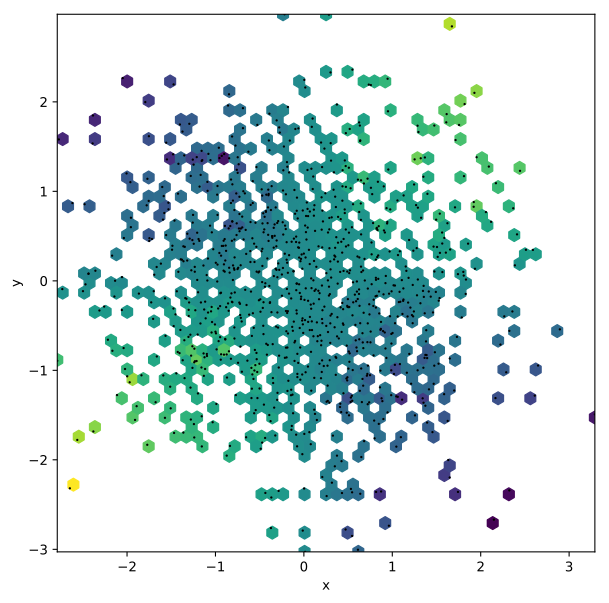# Hextiles¶

#### Title: HexTiles Element¶

Dependencies Matplotlib

Backends Matplotlib, Bokeh

In :
import numpy as np
import holoviews as hv
from holoviews import opts

hv.extension('matplotlib')
hv.output(fig='svg', size=200)HexTiles provide a representation of the data as a bivariate histogram useful for visualizing the structure in larger datasets. The HexTiles are computed by tesselating the x-, y-ranges of the data, storing the number of points falling in each hexagonal bin. By default HexTiles simply counts the data in each bin but it also supports weighted aggregations. Currently only linearly spaced bins are supported when using the bokeh backend.

As a simple example we will generate 100,000 normally distributed points and plot them using HexTiles:

In :
np.random.seed(44)
hex_tiles = hv.HexTiles(np.random.randn(100000, 2))
hex_tiles.opts(colorbar=True)

Out:Note that the hover shows a Count by default, representing the number of points falling within each bin.

It is also possible to provide additional value dimensions and define an aggregator to aggregate by value. If multiple value dimensions are defined the dimension to be colormapped may be defined using the size. Note however that multiple value dimensions are allowed and will be displayed in the hover tooltip.

In :
xs, ys = np.random.randn(2, 1000)
hex_with_values = hv.HexTiles((xs, ys, xs*(ys/2.), (xs**2)*ys), vdims=['Values', 'Hover Values'])
points = hv.Points(hex_with_values)

(hex_with_values * points).opts(
opts.HexTiles(aggregator=np.sum),
opts.Points(s=3, color='black'))

Out:By default HexTiles do not plot bins that do not contain any data but using the min_count option it is possible to plot bins with zero values as well or alternatively set a higher cutoff. Using this options can result in a more visually appealing plot particularly when overlaid with other elements.

Here we will plot the same distributions as above and overlay a Bivariate plot of the same data:

In :
x, y = np.hstack([np.random.randn(2, 10000), np.random.randn(2, 10000)*0.8+2])
hex_two_distributions = hv.HexTiles((x, y))
bivariate = hv.Bivariate((x, y))

(hex_two_distributions * bivariate).opts(
opts.Bivariate(linewidth=3, show_legend=False),
opts.HexTiles(min_count=0))

Out: